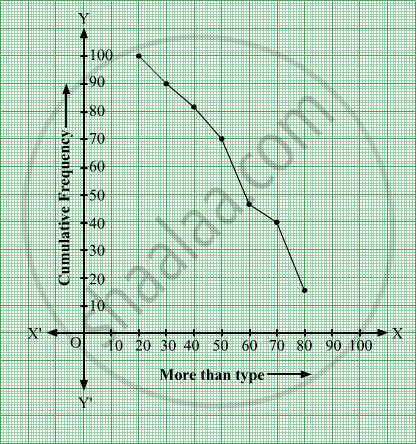# Change the following distribution to a 'more than type' distribution. Hence draw the 'more than type' ogive for this distribution. - Mathematics

Graph

Change the following distribution to a 'more than type' distribution. Hence draw the 'more than type' ogive for this distribution.

 Class interval: 20−30 30−40 40−50 50−60 60−70 70−80 80−90 Frequency: 10 8 12 24 6 25 15

#### Solution

 Class interval Cumulative Frequency More than 20 100 More than 30 90 More than 40 82 More than 50 70 More than 60 46 More than 70 40 More than 80 15Is there an error in this question or solution?